Model Response Variable Distribution Canonical Link Function Traditional Linear Model continuous Normal identity, g(μ) = μ Logistic Regression a count or a binary random variable Binomial logit,Poisson Regression in Log Linear Model a count Poisson log, g(μ) = log(μ) Exponential Regression positive continuous Exponential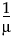JMP fits a generalized linear model to the data by maximum likelihood estimation of the parameter vector. There is, in general, no closed form solution for the maximum likelihood estimates of the parameters. JMP estimates the parameters of the model numerically through an iterative fitting process. The dispersion parameter φ is also estimated by dividing the Pearson goodness-of-fit statistic by its degrees of freedom. Covariances, standard errors, and confidence limits are computed for the estimated parameters based on the asymptotic normality of maximum likelihood estimators.
identity: g(μ) = μ
probit: g(μ) = Φ-1(μ), where Φ is the standard normal cumulative distribution function
log: g(μ) = log(μ)
reciprocal: g(μ) =normal: V(μ) = 1
binomial (proportion): V(μ) = μ(1 – μ)
Poisson: V(μ) = μ
When you select Binomial as the distribution, the response variable must be specified in one of the following ways:
An important aspect of generalized linear modeling is the selection of explanatory variables in the model. Changes in goodness-of-fit statistics are often used to evaluate the contribution of subsets of explanatory variables to a particular model. The deviance, defined to be twice the difference between the maximum attainable log likelihood and the log likelihood at the maximum likelihood estimates of the regression parameters, is often used as a measure of goodness of fit. The maximum attainable log likelihood is achieved with a model that has a parameter for every observation. The following table displays the deviance for each of the probability distributions available in JMP.
 Distribution Deviance normal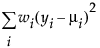Poisson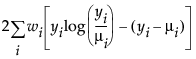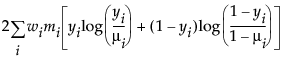exponential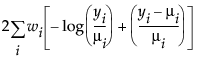In the binomial case, yi = ri /mi, where ri is a binomial count and mi is the binomial number of trials parameter

where yi is the ith response, μi is the corresponding predicted mean, V(μi) is the variance function, and wi is a known weight for the ith observation. If no weight is known, wi = 1 for all observations.